# Find number of times a string occurs as a subsequence

Here, we are going to learn the solution to find number of times a string occurs as a subsequence – which is a popular interview question based on dynamic programming.
Submitted by Radib Kar, on February 15, 2020

Description:

It's a popular interview question based of dynamic programming which has been already featured in Accolite, Amazon.

Problem statement:

Given two strings S and T, find the number of times the second string occurs in the first string, whether continuous or discontinuous as subsequence.

```    Input:
String S: "iloveincludehelp"
String T: "il"

Output:
5
```

Explanation:

The first string is,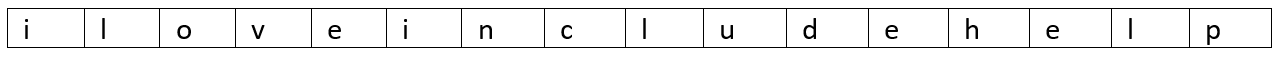The second string is "il"

First occurrence: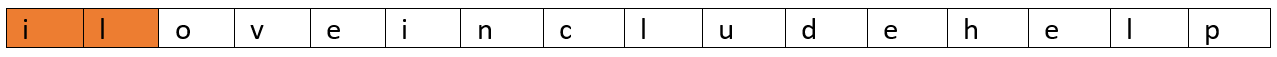Second occurrence: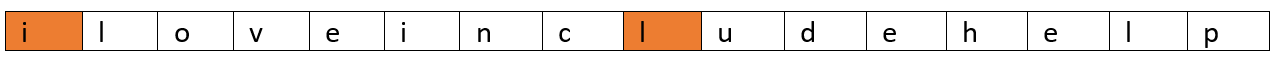Third occurrence: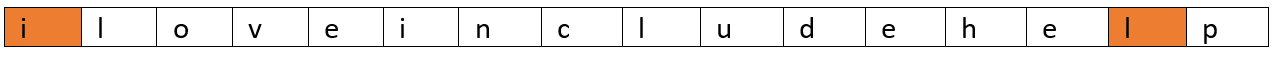Fouth occurrence: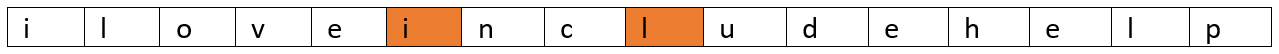Fifth occurrence: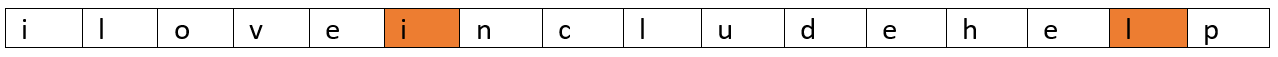So, total distinct occurrences are 5.

Solution Approach:

First, we discuss the recursive solution and then we will convert it to dynamic programming.

Prerequisite:

```    string s: the first string
string t: the second string
starts: start point of the first string
srartt: start point of the second string
m : length of first string
n : length of second string
```

How, how can we generate a recursive relation?

Say,

```    starts=i where i<m and i>=0 & start=j where j<n and j>=0
```

Say,

1. s[starts] = t[start] that means both have same character,
Now we have to option,
1. Check for starts+1, startt+1 which means we are looking for the same occurrence, we want to check for other characters as well.
2. Check for starts+1, startt which means we are looking for another different occurrence.
2. s[starts] != t[start]
Now we have only one option which is check for starts+1, startt as we need to look for different occurrence only.
```    Function distinctOccurence(string s,string t,int starts,int startt,int m,int n)
if startt==n   //enter substring is matched
return 1;

if starts==m         //enter string has been searched with out match
return 0;

if(s[starts]!=t[startt])
//only one option as we discussed
return distinctOccurence(s,t,starts+1,startt,m,n);
else
//both the options as we discussed
return distinctOccurence(s,t,starts+1,startt+1,m,n) + distinctOccurence(s,t,starts+1,startt,m,n);
```

The above recursion will generate many overlapping subproblems and hence we need to use dynamic programming. (I would recommend to take two short string and try doing by your hand and draw the recursion tree to understand how recursion is working).

Let's convert the recursion to DP.

```    Step1: initialize DP table
int dp[m+1][n+1];

Step2: convert step1 of recursive function
for i=0 to n
dp[i]=0;

Step3: convert step2 of recursive function
for i=0 to m
dp[i]=1;

Step4:   Fill the DP table which is similar to step3 of the recursion function
for i=1 to m
for j=1 to n
if s[i-1]==t[j-1]
dp[i][j]=dp[i-1][j]+dp[i-1][j-1]
else
dp[i][j]=dp[i-1][j]
end for
end for

Step5: return dp[m][n] which is the result.
```

C++ Implementation:

```#include <bits/stdc++.h>

using namespace std;

int distinctOccurence(string s, string t, int starts, int startt, int m, int n) {
//note argument k,l  are of no use here
//initialize dp table
int dp[m + 1][n + 1];

//base cases
for (int i = 0; i <= n; i++)
dp[i] = 0;

for (int i = 0; i <= m; i++)
dp[i] = 1;

//fill the dp table
for (int i = 1; i <= m; i++) {
for (int j = 1; j <= n; j++) {
if (s[i - 1] == t[j - 1])
dp[i][j] = dp[i - 1][j] + dp[i - 1][j - 1];
else
dp[i][j] = dp[i - 1][j];
}
}

return dp[m][n];
}

int main() {
int n, m;

string s1, s2;
cout << "Enter the main string:\n";
cin >> s1;
cout << "Enter the substring:\n";
cin >> s2;
m = s1.length();
n = s2.length();
cout << s2 << " has " << distinctOccurence(s1, s2, 0, 0, m, n) << " times different occurences in " << s1 << endl;

return 0;
}
```

Output

```Enter the main string:
iloveincludehelp
Enter the substring:
il
il has 5 times different occurences in iloveincludehelp
```